• 空间注意力机制代码
万次阅读 热门讨论
2022-03-18 12:57:17

各位同学好，今天和大家分享一下attention注意力机制在CNN卷积神经网络中的应用，重点介绍三种注意力机制，及其代码复现。

在我之前的神经网络专栏的文章中也使用到过注意力机制，比如在MobileNetV3、EfficientNet网络中都是用了SE注意力机制，感兴趣的可以看一下：https://blog.csdn.net/dgvv4/category_11517910.html。那么今天就和大家来聊一聊注意力机制。

# 1. 引言

注意力机制源于对人类视觉的研究。在认知科学中，由于信息处理的瓶颈，人类会选择性地关注所有信息中的一部分，同时忽略其他可见信息。为了合理利用有限的视觉信息处理资源，人类需要选择视觉区域中的特定部分，然后重点关注它。

注意力机制没有严格的数学定义，例如传统的局部图像特征提取、滑动窗口方法等都可以看作是一种注意力机制。在神经网络中，注意力机制通常是一个额外的神经网络，能够硬性选择输入的某些部分，或者给输入的不同部分分配不同的权重注意力机制能够从大量的信息中筛选出重要的信息。

在神经网络中引入注意力机制有很多种方法，以卷积神经网络为例，可以在空间维度增加引入注意力机制，也可以在通道维度增加注意力机制（SE），当然也有混合维度（CBAM）即空间维度和通道维度增加注意力机制。

# 2. SENet注意力机制

## 2.1 方法介绍

SE注意力机制（Squeeze-and-Excitation Networks）在通道维度增加注意力机制，关键操作是squeeze和excitation。

通过自动学习的方式，即使用另外一个新的神经网络获取到特征图的每个通道的重要程度，然后用这个重要程度去给每个特征赋予一个权重值，从而让神经网络重点关注某些特征通道提升对当前任务有用的特征图的通道，并抑制对当前任务用处不大的特征通道。

如下图所示，在输入SE注意力机制之前（左侧白图C2），特征图的每个通道的重要程度都是一样的，通过SENet之后（右侧彩图C2），不同颜色代表不同的权重，使每个特征通道的重要性变得不一样了，使神经网络重点关注某些权重值大的通道。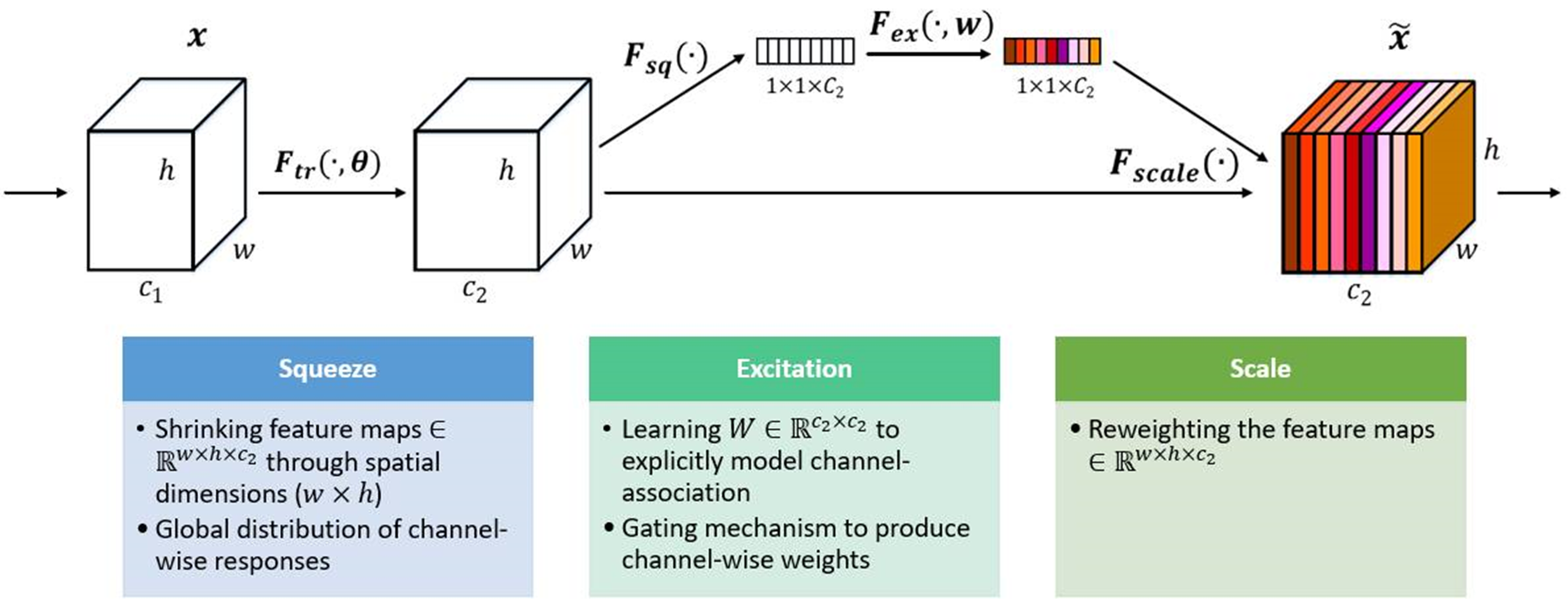## 2.2 实现过程：

（1）Squeeze（Fsq）：通过全局平均池化将每个通道的二维特征（H*W）压缩为1个实数，将特征图从 [h, w, c] ==> [1,1,c]

（2）excitation（Fex）给每个特征通道生成一个权重值，论文中通过两个全连接层构建通道间的相关性，输出的权重值数目和输入特征图的通道数相同。[1,1,c] ==> [1,1,c]

（3）Scale（Fscale）：将前面得到的归一化权重加权到每个通道的特征上论文中使用的是乘法，逐通道乘以权重系数。[h,w,c]*[1,1,c] ==> [h,w,c]

下面我用EfficientNet中的SE注意力机制来说明一下这个流程。

squeeze操作：特征图经过全局平均池化，将特征图压缩成特征向量[1,1,c]

excitation操作：FC1层+Swish激活+FC2层+Sigmoid激活。通过全连接层（FC1），将特征图向量的通道维度降低为原来的1/r，即[1,1,c*1/r]；然后经过Swish激活函数；再通过一个全连接层（FC2），将特征图向量的特征图上升回原来[1,1,c]；然后经过sigmoid函数转化为一个0-1之间的归一化权重向量。

scale操作：将归一化权重和原输入特征图逐通道相乘，生成加权后的特征图。### 小节：

（1）SENet的核心思想是通过全连接网络根据loss损失来自动学习特征权重，而不是直接根据特征通道的数值分配来判断，使有效的特征通道的权重大。当然SE注意力机制不可避免的增加了一些参数和计算量，但性价比还是挺高的。

（2）论文认为excitation操作中使用两个全连接层相比直接使用一个全连接层，它的好处在于，具有更多的非线性，可以更好地拟合通道间的复杂关联。

## 2.3 代码复现

import tensorflow as tf
from tensorflow.keras import layers, Model, Input

# se注意力机制
def se_block(inputs, ratio=4):  # ratio代表第一个全连接层下降通道数的系数

# 获取输入特征图的通道数
in_channel = inputs.shape[-1]

# 全局平均池化[h,w,c]==>[None,c]
x = layers.GlobalAveragePooling2D()(inputs)

# [None,c]==>[1,1,c]
x = layers.Reshape(target_shape=(1,1,in_channel))(x)

# [1,1,c]==>[1,1,c/4]
x = layers.Dense(in_channel//ratio)(x)  # 全连接下降通道数

# relu激活
x = tf.nn.relu(x)

# [1,1,c/4]==>[1,1,c]
x = layers.Dense(in_channel)(x)  # 全连接上升通道数

# sigmoid激活，权重归一化
x = tf.nn.sigmoid(x)

# [h,w,c]*[1,1,c]==>[h,w,c]
outputs = layers.multiply([inputs, x])  # 归一化权重和原输入特征图逐通道相乘

return outputs

# 测试SE注意力机制
if __name__ == '__main__':

# 构建输入
inputs = Input([56,56,24])

x = se_block(inputs)  # 接收SE返回值

model = Model(inputs, x)  # 构建网络模型

print(x.shape)  # (None, 56, 56, 24)
model.summary()  # 输出SE模块的结构

查看SE模块的结构框架

Model: "model"
__________________________________________________________________________________________________
Layer (type)                    Output Shape         Param #     Connected to
==================================================================================================
input_1 (InputLayer)            [(None, 56, 56, 24)] 0
__________________________________________________________________________________________________
global_average_pooling2d (Globa (None, 24)           0           input_1
__________________________________________________________________________________________________
reshape (Reshape)               (None, 1, 1, 24)     0           global_average_pooling2d
__________________________________________________________________________________________________
dense (Dense)                   (None, 1, 1, 6)      150         reshape
__________________________________________________________________________________________________
tf.nn.relu (TFOpLambda)         (None, 1, 1, 6)      0           dense
__________________________________________________________________________________________________
dense_1 (Dense)                 (None, 1, 1, 24)     168         tf.nn.relu
__________________________________________________________________________________________________
tf.math.sigmoid (TFOpLambda)    (None, 1, 1, 24)     0           dense_1
__________________________________________________________________________________________________
multiply (Multiply)             (None, 56, 56, 24)   0           input_1
tf.math.sigmoid
==================================================================================================
Total params: 318
Trainable params: 318
Non-trainable params: 0
__________________________________________________________________________________________________

# 3. ECANet注意力机制

## 3.1 方法介绍

ECANet是通道注意力机制的一种实现形式，ECANet可以看做是SENet的改进版。

作者表明SENet中的降维会给通道注意力机制带来副作用，并且捕获所有通道之间的依存关系是效率不高的且是不必要的。

ECA注意力机制模块直接在全局平均池化层之后使用1x1卷积层，去除了全连接层。该模块避免了维度缩减，并有效捕获了跨通道交互。并且ECA只涉及少数参数就能达到很好的效果。

ECANet通过一维卷积 layers.Conv1D来完成跨通道间的信息交互，卷积核的大小通过一个函数来自适应变化，使得通道数较大的层可以更多地进行跨通道交互。自适应函数为：，其中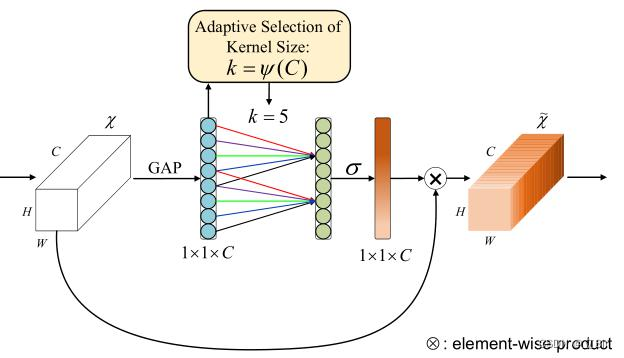## 3.2 实现过程

（1）将输入特征图经过全局平均池化，特征图从[h,w,c]的矩阵变成[1,1,c]的向量

（2）计算得到自适应的一维卷积核大小kernel_size

（3）将kernel_size用于一维卷积中，得到对于特征图的每个通道的权重

（4）将归一化权重和原输入特征图逐通道相乘，生成加权后的特征图

## 3.3 代码实现

import tensorflow as tf
from tensorflow import keras
from tensorflow.keras import Model, layers
import math

def eca_block(inputs, b=1, gama=2):

# 输入特征图的通道数
in_channel = inputs.shape[-1]

# 根据公式计算自适应卷积核大小
kernel_size = int(abs((math.log(in_channel, 2) + b) / gama))

# 如果卷积核大小是偶数，就使用它
if kernel_size % 2:
kernel_size = kernel_size

# 如果卷积核大小是奇数就变成偶数
else:
kernel_size = kernel_size + 1

# [h,w,c]==>[None,c] 全局平均池化
x = layers.GlobalAveragePooling2D()(inputs)

# [None,c]==>[c,1]
x = layers.Reshape(target_shape=(in_channel, 1))(x)

# [c,1]==>[c,1]
x = layers.Conv1D(filters=1, kernel_size=kernel_size, padding='same', use_bias=False)(x)

# sigmoid激活
x = tf.nn.sigmoid(x)

# [c,1]==>[1,1,c]
x = layers.Reshape((1,1,in_channel))(x)

# 结果和输入相乘
outputs = layers.multiply([inputs, x])

return outputs

# 验证ECA注意力机制
if __name__ == '__main__':

# 构造输入层
inputs = keras.Input(shape=[26,26,512])

x = eca_block(inputs)  # 接收ECA输出结果

model = Model(inputs, x)  # 构造模型
model.summary()  # 查看网络架构

查看ECA模块，和SENet相比大大减少了参数量，参数量等于一维卷积的kernel_size的大小

Model: "model_1"
__________________________________________________________________________________________________
Layer (type)                    Output Shape         Param #     Connected to
==================================================================================================
input_2 (InputLayer)            [(None, 26, 26, 512) 0
__________________________________________________________________________________________________
global_average_pooling2d_1 (Glo (None, 512)          0           input_2
__________________________________________________________________________________________________
reshape_1 (Reshape)             (None, 512, 1)       0           global_average_pooling2d_1
__________________________________________________________________________________________________
conv1d (Conv1D)                 (None, 512, 1)       5           reshape_1
__________________________________________________________________________________________________
tf.math.sigmoid_1 (TFOpLambda)  (None, 512, 1)       0           conv1d
__________________________________________________________________________________________________
reshape_2 (Reshape)             (None, 1, 1, 512)    0           tf.math.sigmoid_1
__________________________________________________________________________________________________
multiply_1 (Multiply)           (None, 26, 26, 512)  0           input_2
reshape_2
==================================================================================================
Total params: 5
Trainable params: 5
Non-trainable params: 0
__________________________________________________________________________________________________tensorflow 深度学习 神经网络
更多相关内容
• ## 【python】注意力机制代码

万次阅读 多人点赞 2021-06-08 17:48:52
梳理目前主流的注意力机制代码，目前以pytorch为例。 说明： 特征图维度的组织形式为：（batch,channel,height,width） 1. 正文 1.1 SEBlock 2017 考虑通道间的注意力之间的关系，在通道上加入注意力机制 论文：...

every blog every motto: You can do more than you think.

# 0. 前言

梳理目前主流的注意力机制代码，目前以pytorch为例。
说明：

1. 特征图维度的组织形式为：（batch,channel,height,width）
2. 后续增加

# 1. 正文

## 1.1 SEBlock 2017

考虑通道间的注意力之间的关系，在通道上加入注意力机制
论文：https://arxiv.org/abs/1709.01507
代码：https://github.com/hujie-frank/SENet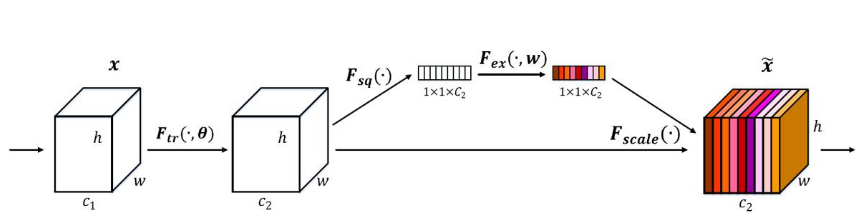对于输入特征图C2，其后加上SE注意力模块

### 1.1.1 步骤

主要分三步：

1. squeeze，对空间维度进行压缩，代码上即利用全局平均池化将每个通道平均成一个值，该值具有全局感受野。（通俗理解：一个通道理解为一个大饼，多个通道就是多个大饼垒在一起。全局平均池化即将一个大饼平均成一个点，整体看，类似一个垒起来的色子）
维度变化：（2,512,8,8）-> (2,512,1,1) ==> (2,512)
2. excitation, 利用权重学习上面各通道间的相关性，代码实现有全连接和卷积核为1的卷积操作两种方式。
维度变化：（2,512）-> (2,512//reducation)->(2,512) ==>(2,512,1,1)
说明： 该过程先降维在升维，降维倍数由reducation参数决定，降低网络的计算量，其中的激活函数增加了网络的非线性。
3. scale: 通过上面excitation的操作输出了每个通道的重要性，在通过乘法加权操作乘以输入数据C2，从而提升重要特征，抑制不重要特征。
维度变化：（2,512,8,8）*(2,512,1,1) -> (2,512,16,16)

小结： 即输入维度为（2,512,8,8），输出维度为：（2,512,8,8）
说明： 上述步骤中的“->”表示维度变化方向，“==>”表示通过view方法改变了维度。

### 1.1.2 更加清晰的理解图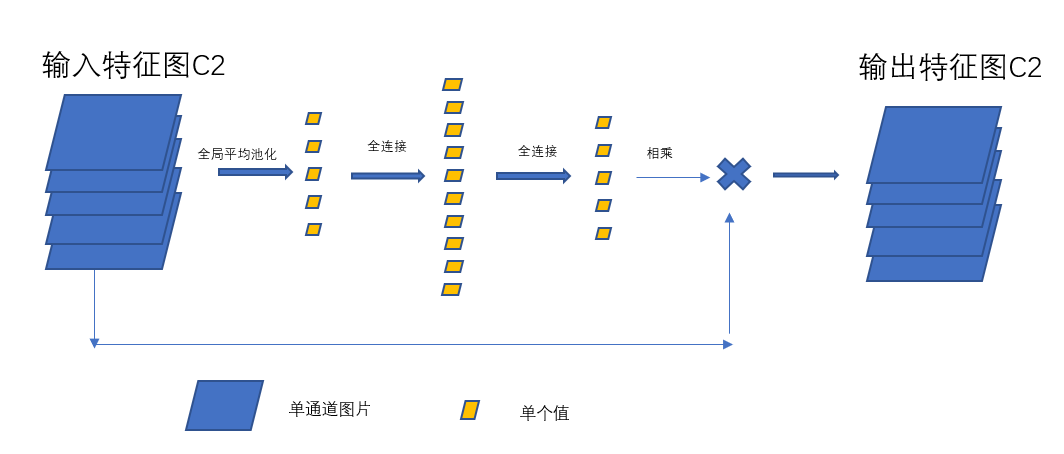说明：

1. 全连接和1 × 1的卷积效果类似，上图显示为全连接，亦可为1*1的卷积，下同，不赘述。
2. 激活函数位置见代码，下同。

### 1.1.3 代码

#### 1. pytorch

class SELayer(nn.Module):
def __init__(self, channel, reduction=4):
""" SE注意力机制,输入x。输入输出特征图不变
1.squeeze: 全局池化 (batch,channel,height,width) -> (batch,channel,1,1) ==> (batch,channel)
2.excitaton: 全连接or卷积核为1的卷积(batch,channel)->(batch,channel//reduction)-> (batch,channel) ==> (batch,channel,1,1) 输出y
3.scale: 完成对通道维度上原始特征的标定 y = x*y 输出维度和输入维度相同

:param channel: 输入特征图的通道数
:param reduction: 特征图通道的降低倍数
"""
super(SELayer, self).__init__()
# 自适应全局平均池化,即，每个通道进行平均池化，使输出特征图长宽为1

# 全连接的excitation
self.fc = nn.Sequential(
nn.Linear(channel, channel // reduction),
nn.ReLU(inplace=True),
nn.Linear(channel // reduction, channel),
nn.Sigmoid()
)
# 卷积网络的excitation
# 特征图变化：
# (2,512,1,1) -> (2,512,1,1) -> (2,512,1,1)
self.fc2 = nn.Sequential(
nn.Conv2d(channel, channel // reduction, 1, bias=False),
nn.ReLU(inplace=True),
nn.Conv2d(channel // reduction, channel, 1, bias=False),
nn.Sigmoid()
)

def forward(self, x):
# (batch,channel,height,width) (2,512,8,8)
b, c, _, _ = x.size()
# 全局平均池化 (2,512,8,8) -> (2,512,1,1) -> (2,512)
y = self.avg_pool(x).view(b, c)
# (2,512) -> (2,512//reducation) -> (2,512) -> (2,512,1,1)
y = self.fc(y).view(b, c, 1, 1)
# (2,512,8,8)* (2,512,1,1) -> (2,512,8,8)
pro = x * y
return x * y


#### 2. tensorflow/keras

# SEBlock
feature_map_shape = input_x.shape  # input feature map shape
x = tf.reduce_mean(x, [1, 2])  # reduce along axis 1 and 2 ,height,width,
x = Dense(feature_map_shape[-1] / 16, activation=tf.nn.relu)(x)  # (batch,channel) -> (batch,channel/16)
x = Dense(feature_map_shape[-1], activation=tf.nn.relu)(x)  # (batch,channel/16) -> (batch,channel)
x = tf.multiply(input_x, x)  # multiply along channel


说明：

1. 当使用全连接时，forward中平均池 (squeeze)，
# 全局平均池化 (2,512,8,8) -> (2,512,1,1) -> (2,512)
y = self.avg_pool(x).view(b, c)

1. 当使用1*1卷积，forward中平均池化（squeeze）,
# 全局平均池化 (2,512,8,8) -> (2,512,1,1)
y = self.avg_pool(x)


## 1.2 CBAM 2018

论文：https://arxiv.org/abs/1807.06521
代码：https://github.com/luuuyi/CBAM.PyTorch
CBAM可以无缝集成任何CNN架构中，开销不大，早期的注意力机制一种。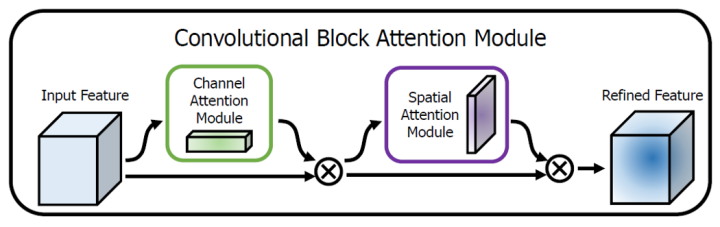实验结果表明：顺序链接比并行连接好，其中通道注意力在前优于空间注意力在前。

### 1.2.1 通道注意力机制

#### 1.2.1.1 概述

通道注意力机制和上面的SEBlock类似，唯一不同的是加了一个最大池化。而后，最大池化和平均池化共用一个多层感知机（mlp）, 再将结果相加和输入特征图进行点乘传入空间注意力机制。
说明： 主要步骤省略，可参考SEBlock和下面代码中的注释。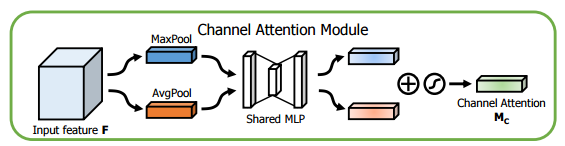#### 1.2.1.2 更加清晰的理解图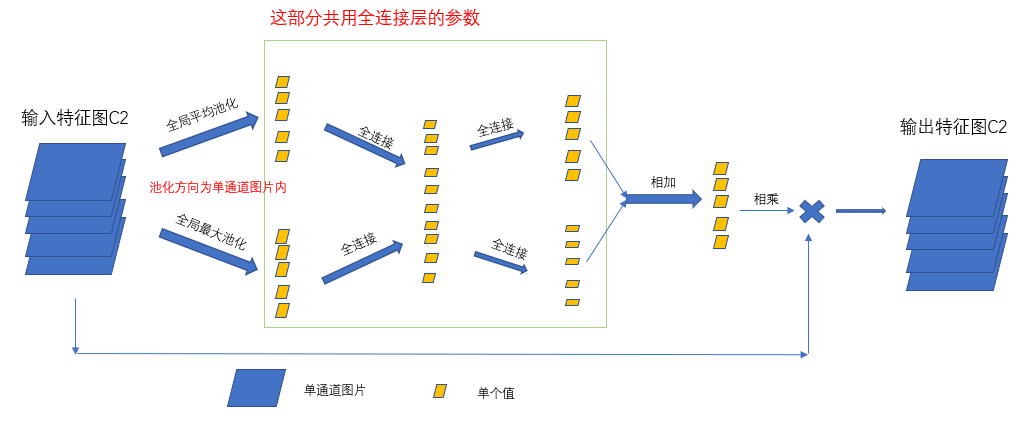#### 1.2.1.3 代码

说明： forward中，为了方便理解，展开书写了，等价于最开始注释了的几行。

class ChannelAttention(nn.Module):
def __init__(self, in_channel, ratio=16):
""" 通道注意力机制 同最大池化和平均池化两路分别提取信息，后共用一个多层感知机mlp,再将二者结合

:param in_channel: 输入通道
:param ratio: 通道降低倍率
"""
super(ChannelAttention, self).__init__()
# 平均池化
# 最大池化

# 通道先降维后恢复到原来的维数
self.fc1 = nn.Conv2d(in_channel, in_channel // ratio, 1, bias=False)
self.relu1 = nn.ReLU()
self.fc2 = nn.Conv2d(in_channel // ratio, in_channel, 1, bias=False)
self.sigmoid = nn.Sigmoid()

def forward(self, x):
# 平均池化
# avg_out = self.fc2(self.relu1(self.fc1(self.avg_pool(x))))
# 最大池化
# max_out = self.fc2(self.relu1(self.fc1(self.max_pool(x))))
# out = avg_out + max_out
# return x*self.sigmoid(out)

# 平均池化一支 (2,512,8,8) -> (2,512,1,1) -> (2,512/ration,1,1) -> (2,512,1,1)
# (2,512,8,8) -> (2,512,1,1)
avg = self.avg_pool(x)
# 多层感知机mlp (2,512,8,8) -> (2,512,1,1) -> (2,512/ration,1,1) -> (2,512,1,1)
# (2,512,1,1) -> (2,512/ratio,1,1)
avg = self.fc1(avg)
avg = self.relu1(avg)
# (2,512/ratio,1,1) -> (2,512,1,1)
avg_out = self.fc2(avg)

# 最大池化一支
# (2,512,8,8) -> (2,512,1,1)
max = self.max_pool(x)
# 多层感知机
# (2,512,1,1) -> (2,512/ratio,1,1)
max = self.fc1(max)
max = self.relu1(max)
# (2,512/ratio,1,1) -> (2,512,1,1)
max_out = self.fc2(max)

# (2,512,1,1) + (2,512,1,1) -> (2,512,1,1)
out = avg_out + max_out
return x*self.sigmoid(out)


### 1.2.2 空间注意力机制

#### 1.2.2.1 概述

将通道注意力机制的的结果作为新的输入特征图，主要步骤：

1. 输入特征图，经过最大池化,平均池化（通道维度压缩，与前面的通道注意力机制不同）
维度变化：（2,512,8，8 ） -> (2,1,8,8)
2. 将最大池化和平均池化在通道方向上合并
维度变化：（2,1,8,8）+ (2,1,8,8) -> (2,2,8,8)
3.经过卷积，通道变为1,再经过激活函数
维度变化：（2,2，8,8）-> (2,1,8,8)
3. 和输入特征图点乘
维度变化：(2,512,8,8) * (2,1,8,8) -> (2,512,8,8)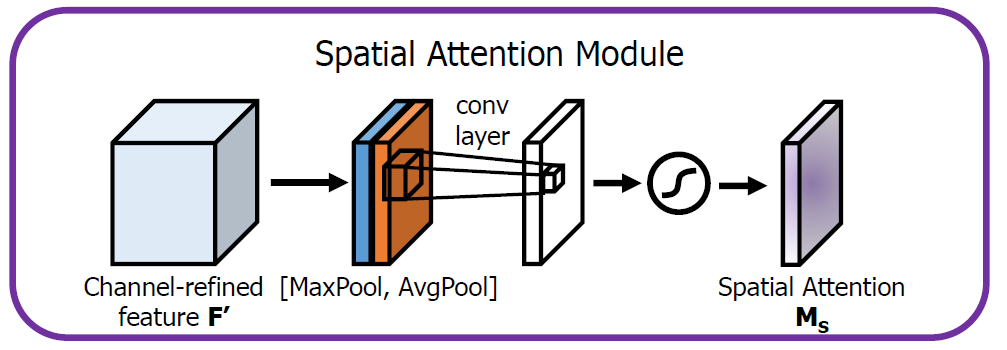#### 1.2.2.2 更加清晰的理解图

说明： 下面两种图例其实是一个意思，即，单通道特征图，为了看起来更加清晰用了两种颜色。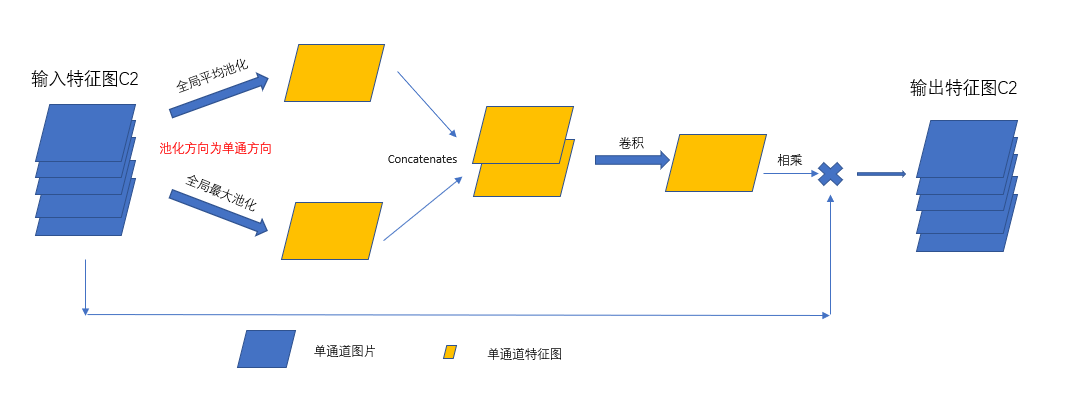#### 1.2.2.3 代码

class SpatialAttention(nn.Module):
def __init__(self, kernel_size=7):
""" 空间注意力机制 将通道维度通过最大池化和平均池化进行压缩，然后合并，再经过卷积和激活函数，结果和输入特征图点乘

:param kernel_size: 卷积核大小
"""
super(SpatialAttention, self).__init__()

assert kernel_size in (3, 7), 'kernel size must be 3 or 7'
padding = 3 if kernel_size == 7 else 1

self.sigmoid = nn.Sigmoid()

def forward(self, x):
print('x shape', x.shape)
# (2,512,8,8) -> (2,1,8,8)
avg_out = torch.mean(x, dim=1, keepdim=True)
# (2,512,8,8) -> (2,1,8,8)
max_out, _ = torch.max(x, dim=1, keepdim=True)
# (2,1,8,8) + (2,1,8,8) -> (2,2,8,8)
cat = torch.cat([avg_out, max_out], dim=1)
# (2,2,8,8) -> (2,1,8,8)
out = self.conv1(cat)
return x * self.sigmoid(out)


展开全文python pytorch 深度学习
• 神经网络学习小记录65——Tensorflow2 图像处理中注意力机制的解析与代码详解学习前言什么是注意力机制代码下载注意力机制的实现方式1、SENet的实现2、CBAM的实现3、ECA的实现注意力机制的应用 学习前言 注意力机制...

# 学习前言

注意力机制是一个非常有效的trick，注意力机制的实现方式有许多，我们一起来学习一下。# 什么是注意力机制

注意力机制是深度学习常用的一个小技巧，它有多种多样的实现形式，尽管实现方式多样，但是每一种注意力机制的实现的核心都是类似的，就是注意力

注意力机制的核心重点就是让网络关注到它更需要关注的地方

当我们使用卷积神经网络去处理图片的时候，我们会更希望卷积神经网络去注意应该注意的地方，而不是什么都关注，我们不可能手动去调节需要注意的地方，这个时候，如何让卷积神经网络去自适应的注意重要的物体变得极为重要。

注意力机制就是实现网络自适应注意的一个方式。

一般而言，注意力机制可以分为通道注意力机制，空间注意力机制，以及二者的结合。# 代码下载

Github源码下载地址为：
https://github.com/bubbliiiing/yolov4-tiny-tf2
复制该路径到地址栏跳转。

# 注意力机制的实现方式

在深度学习中，常见的注意力机制的实现方式有SENet，CBAM，ECA等等。

## 1、SENet的实现

SENet是通道注意力机制的典型实现。
2017年提出的SENet是最后一届ImageNet竞赛的冠军，其实现示意图如下所示，对于输入进来的特征层，我们关注其每一个通道的权重，对于SENet而言，其重点是获得输入进来的特征层，每一个通道的权值。利用SENet，我们可以让网络关注它最需要关注的通道。

其具体实现方式就是：
1、对输入进来的特征层进行全局平均池化
2、然后进行两次全连接，第一次全连接神经元个数较少，第二次全连接神经元个数和输入特征层相同
3、在完成两次全连接后，我们再取一次Sigmoid将值固定到0-1之间，此时我们获得了输入特征层每一个通道的权值（0-1之间）。
4、在获得这个权值后，我们将这个权值乘上原输入特征层即可。实现代码如下：

def se_block(input_feature, ratio=16, name=""):
channel = input_feature._keras_shape[-1]

se_feature = GlobalAveragePooling2D()(input_feature)
se_feature = Reshape((1, 1, channel))(se_feature)

se_feature = Dense(channel // ratio,
activation='relu',
kernel_initializer='he_normal',
use_bias=False,
bias_initializer='zeros',
name = "se_block_one_"+str(name))(se_feature)

se_feature = Dense(channel,
kernel_initializer='he_normal',
use_bias=False,
bias_initializer='zeros',
name = "se_block_two_"+str(name))(se_feature)
se_feature = Activation('sigmoid')(se_feature)

se_feature = multiply([input_feature, se_feature])
return se_feature


## 2、CBAM的实现

CBAM将通道注意力机制和空间注意力机制进行一个结合，相比于SENet只关注通道的注意力机制可以取得更好的效果。其实现示意图如下所示，CBAM会对输入进来的特征层，分别进行通道注意力机制的处理和空间注意力机制的处理下图是通道注意力机制和空间注意力机制的具体实现方式：
图像的上半部分为通道注意力机制通道注意力机制的实现可以分为两个部分，我们会对输入进来的单个特征层，分别进行全局平均池化全局最大池化。之后对平均池化最大池化的结果，利用共享的全连接层进行处理，我们会对处理后的两个结果进行相加，然后取一个sigmoid，此时我们获得了输入特征层每一个通道的权值（0-1之间）。在获得这个权值后，我们将这个权值乘上原输入特征层即可。

图像的下半部分为空间注意力机制，我们会对输入进来的特征层，在每一个特征点的通道上取最大值和平均值。之后将这两个结果进行一个堆叠，利用一次通道数为1的卷积调整通道数，然后取一个sigmoid，此时我们获得了输入特征层每一个特征点的权值（0-1之间）。在获得这个权值后，我们将这个权值乘上原输入特征层即可。实现代码如下：

def channel_attention(input_feature, ratio=8, name=""):

channel = input_feature._keras_shape[-1]

shared_layer_one = Dense(channel//ratio,
activation='relu',
kernel_initializer='he_normal',
use_bias=False,
bias_initializer='zeros',
name = "channel_attention_shared_one_"+str(name))
shared_layer_two = Dense(channel,
kernel_initializer='he_normal',
use_bias=False,
bias_initializer='zeros',
name = "channel_attention_shared_two_"+str(name))

avg_pool = GlobalAveragePooling2D()(input_feature)
max_pool = GlobalMaxPooling2D()(input_feature)

avg_pool = Reshape((1,1,channel))(avg_pool)
max_pool = Reshape((1,1,channel))(max_pool)

avg_pool = shared_layer_one(avg_pool)
max_pool = shared_layer_one(max_pool)

avg_pool = shared_layer_two(avg_pool)
max_pool = shared_layer_two(max_pool)

cbam_feature = Activation('sigmoid')(cbam_feature)

return multiply([input_feature, cbam_feature])

def spatial_attention(input_feature, name=""):
kernel_size = 7

cbam_feature = input_feature

avg_pool = Lambda(lambda x: K.mean(x, axis=3, keepdims=True))(cbam_feature)
max_pool = Lambda(lambda x: K.max(x, axis=3, keepdims=True))(cbam_feature)
concat = Concatenate(axis=3)([avg_pool, max_pool])

cbam_feature = Conv2D(filters = 1,
kernel_size=kernel_size,
strides=1,
kernel_initializer='he_normal',
use_bias=False,
name = "spatial_attention_"+str(name))(concat)
cbam_feature = Activation('sigmoid')(cbam_feature)

return multiply([input_feature, cbam_feature])

def cbam_block(cbam_feature, ratio=8, name=""):
cbam_feature = channel_attention(cbam_feature, ratio, name=name)
cbam_feature = spatial_attention(cbam_feature, name=name)
return cbam_feature


## 3、ECA的实现

ECANet是也是通道注意力机制的一种实现形式。ECANet可以看作是SENet的改进版。
ECANet的作者认为SENet对通道注意力机制的预测带来了副作用捕获所有通道的依赖关系是低效并且是不必要的
在ECANet的论文中，作者认为卷积具有良好的跨通道信息获取能力

ECA模块的思想是非常简单的，它去除了原来SE模块中的全连接层，直接在全局平均池化之后的特征上通过一个1D卷积进行学习。

既然使用到了1D卷积，那么1D卷积的卷积核大小的选择就变得非常重要了，了解过卷积原理的同学很快就可以明白，1D卷积的卷积核大小会影响注意力机制每个权重的计算要考虑的通道数量。用更专业的名词就是跨通道交互的覆盖率

如下图所示，左图是常规的SE模块，右图是ECA模块。ECA模块用1D卷积替换两次全连接。实现代码如下：

def eca_block(input_feature, b=1, gamma=2, name=""):
channel = input_feature._keras_shape[-1]
kernel_size = int(abs((math.log(channel, 2) + b) / gamma))
kernel_size = kernel_size if kernel_size % 2 else kernel_size + 1

avg_pool = GlobalAveragePooling2D()(input_feature)

x = Reshape((-1,1))(avg_pool)
x = Conv1D(1, kernel_size=kernel_size, padding="same", name = "eca_layer_"+str(name), use_bias=False,)(x)
x = Activation('sigmoid')(x)
x = Reshape((1, 1, -1))(x)

output = multiply([input_feature,x])
return output


# 注意力机制的应用

注意力机制是一个即插即用的模块，理论上可以放在任何一个特征层后面，可以放在主干网络，也可以放在加强特征提取网络。

由于放置在主干会导致网络的预训练权重无法使用，本文以YoloV4-tiny为例，将注意力机制应用加强特征提取网络上。

如下图所示，我们在主干网络提取出来的两个有效特征层上增加了注意力机制，同时对上采样后的结果增加了注意力机制实现代码如下：

attention = [se_block, cbam_block, eca_block]

#---------------------------------------------------#
#   特征层->最后的输出
#---------------------------------------------------#
def yolo_body(input_shape, anchors_mask, num_classes, phi = 0):
inputs = Input(input_shape)
#---------------------------------------------------#
#   生成CSPdarknet53_tiny的主干模型
#   feat1的shape为26,26,256
#   feat2的shape为13,13,512
#---------------------------------------------------#
feat1, feat2 = darknet_body(inputs)
if phi >= 1 and phi <= 3:
feat1 = attention[phi - 1](feat1, name='feat1')
feat2 = attention[phi - 1](feat2, name='feat2')

# 13,13,512 -> 13,13,256
P5 = DarknetConv2D_BN_Leaky(256, (1,1))(feat2)
# 13,13,256 -> 13,13,512 -> 13,13,255
P5_output = DarknetConv2D_BN_Leaky(512, (3,3))(P5)
P5_output = DarknetConv2D(len(anchors_mask) * (num_classes+5), (1,1))(P5_output)

# 13,13,256 -> 13,13,128 -> 26,26,128
P5_upsample = compose(DarknetConv2D_BN_Leaky(128, (1,1)), UpSampling2D(2))(P5)
if phi >= 1 and phi <= 3:
P5_upsample = attention[phi - 1](P5_upsample, name='P5_upsample')

# 26,26,256 + 26,26,128 -> 26,26,384
P4 = Concatenate()([P5_upsample, feat1])

# 26,26,384 -> 26,26,256 -> 26,26,255
P4_output = DarknetConv2D_BN_Leaky(256, (3,3))(P4)
P4_output = DarknetConv2D(len(anchors_mask) * (num_classes+5), (1,1))(P4_output)

return Model(inputs, [P5_output, P4_output])

展开全文神经网络 图像处理 深度学习 Attention
• 各位同学好，今天和大家分享一下如何使用 Tensorflow 构建 DANet 和 CBAM 混合域注意力机制模型。在之前的文章中我介绍了CNN中的通道注意力机制 SENet 和 ECANet，感兴趣的可以看一下：...

各位同学好，今天和大家分享一下如何使用 Tensorflow 构建 DANetCBAM 混合域注意力机制模型。在之前的文章中我介绍了CNN中的通道注意力机制 SENet 和 ECANet，感兴趣的可以看一下：https://blog.csdn.net/dgvv4/article/details/123572065

# 1. 注意力机制介绍

注意力机制本质上是一种资源分配机制，它可以根据关注目标的重要性程度改变资源分配方式，使资源更多的向attention的对象倾斜。在卷积神经网络中，注意力机制所要分配的资源就是权重参数。在模型训练过程中对于attention的对象分配更多的权重参数，能够提高对于attention对象的特征提取能力。在目标检测任务中添加注意力机制，可以提高模型的表征能力，有效减少无效目标的千扰，提升对关注目标的检测效果，进而提高模型的整体检测准确率。

# 2. CBAM 注意力机制

## 2.1 方法介绍

CBAM注意力机制是由通道注意力机制（channel）和空间注意力机制（spatial）组成。

CNAM注意力机制的优点：

（1）轻量化程度高：CBAM模块内部无大量卷积结构，少量池化层和特征融合操作，这种结构避免了卷积乘法带来的大量计算，使得其模块复杂度低，计算量小。实验证明，在轻量模型上添加CBAM模块能够带来稳定的性能提升，相较于其带来的少量的计算量的增加，CBAM的引入具有很局的性价比。

（2）通用性强：其结构特点决定了CBAM的通用性强，可移植性高，主要体现在两方面：一方面，基于池化操作的CBAM模块能够直接嵌入到卷积操作后，这意味着该模块可以添加到诸如VGG的传统神经网络中，同时也可以添加到包含基于shortcut连接的残差结构的网络中，如ResNet50、MobileNetV3；另一方面，CBAM同时适用于目标检测和分类任务，且对于不同数据特征的数据集，在检测或者分类精度上均能取得较好的性能提升。

（3）作用效果佳：传统基于卷积神经网络的注意力机制更多的是关注对通道域的分析，局限于考虑特征图通道之间的作用关系。CBAM从 channel 和 spatial 两个作用域出发，引入空间注意力和通道注意力两个分析维度，实现从通道到空间的顺序注意力结构。空间注意力可使神经网络更加关注图像中对分类起决定作用的像素区域而忽略无关紧要的区域通道注意力则用于处理特征图通道的分配关系，同时对两个维度进行注意力分配增强了注意力机制对模型性能的提升效果。

## 2.2 网络结构

### （1）通道注意力机制代码展示

#（1）通道注意力
def channel_attenstion(inputs, ratio=0.25):
'''ratio代表第一个全连接层下降通道数的倍数'''

channel = inputs.shape[-1]  # 获取输入特征图的通道数

# 分别对输出特征图进行全局最大池化和全局平均池化
# [h,w,c]==>[None,c]
x_max = layers.GlobalMaxPooling2D()(inputs)
x_avg = layers.GlobalAveragePooling2D()(inputs)

# [None,c]==>[1,1,c]
x_max = layers.Reshape([1,1,-1])(x_max)  # -1代表自动寻找通道维度的大小
x_avg = layers.Reshape([1,1,-1])(x_avg)  # 也可以用变量channel代替-1

# 第一个全连接层通道数下降1/4, [1,1,c]==>[1,1,c//4]
x_max = layers.Dense(channel*ratio)(x_max)
x_avg = layers.Dense(channel*ratio)(x_avg)

# relu激活函数
x_max = layers.Activation('relu')(x_max)
x_avg = layers.Activation('relu')(x_avg)

# 第二个全连接层上升通道数, [1,1,c//4]==>[1,1,c]
x_max = layers.Dense(channel)(x_max)
x_avg = layers.Dense(channel)(x_avg)

# 结果在相叠加 [1,1,c]+[1,1,c]==>[1,1,c]

# 经过sigmoid归一化权重
x = tf.nn.sigmoid(x)

# 输入特征图和权重向量相乘，给每个通道赋予权重
x = layers.Multiply()([inputs, x])  # [h,w,c]*[1,1,c]==>[h,w,c]

return x

### （2）空间注意力机制

CBAM中的空间注意力机制模块如下。对通道注意力机制的输出特征图进行空间域的处理。首先，特征图分别经过基于通道维度的最大池化和平均池化将输出的两张特征图在通道维度堆叠 layers.concatenate()。然后使用1*1卷积调整通道数，最后经过sigmoid函数归一化权重。将归一化权重和输入特征度相乘。代码展示

#（2）空间注意力机制
def spatial_attention(inputs):

# 在通道维度上做最大池化和平均池化[b,h,w,c]==>[b,h,w,1]
# keepdims=Fale那么[b,h,w,c]==>[b,h,w]
x_max = tf.reduce_max(inputs, axis=3, keepdims=True)  # 在通道维度求最大值
x_avg = tf.reduce_mean(inputs, axis=3, keepdims=True)  # axis也可以为-1

# 在通道维度上堆叠[b,h,w,2]
x = layers.concatenate([x_max, x_avg])

# 1*1卷积调整通道[b,h,w,1]
x = layers.Conv2D(filters=1, kernel_size=(1,1), strides=1, padding='same')(x)

# sigmoid函数权重归一化
x = tf.nn.sigmoid(x)

# 输入特征图和权重相乘
x = layers.Multiply()([inputs, x])

return x

### （3）总体流程

CBAM的总体流程图如下。输入特征图想经过通道注意力机制，将权重和输入特征图相乘后再送入空间注意力机制，将归一化权重和空间注意力机制的输入特征图相乘，得到最终的特征图。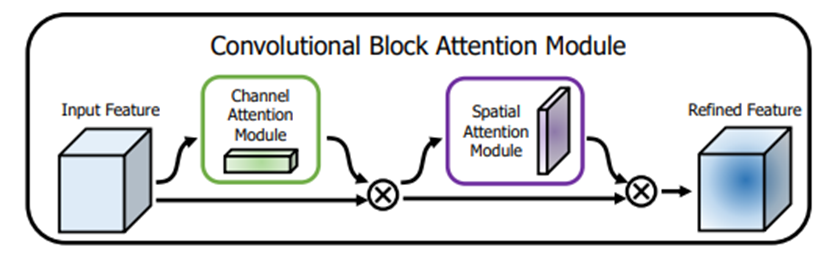完整代码展示

import tensorflow as tf
from tensorflow import keras
from tensorflow.keras import layers, Model

#（1）通道注意力
def channel_attenstion(inputs, ratio=0.25):
'''ratio代表第一个全连接层下降通道数的倍数'''

channel = inputs.shape[-1]  # 获取输入特征图的通道数

# 分别对输出特征图进行全局最大池化和全局平均池化
# [h,w,c]==>[None,c]
x_max = layers.GlobalMaxPooling2D()(inputs)
x_avg = layers.GlobalAveragePooling2D()(inputs)

# [None,c]==>[1,1,c]
x_max = layers.Reshape([1,1,-1])(x_max)  # -1代表自动寻找通道维度的大小
x_avg = layers.Reshape([1,1,-1])(x_avg)  # 也可以用变量channel代替-1

# 第一个全连接层通道数下降1/4, [1,1,c]==>[1,1,c//4]
x_max = layers.Dense(channel*ratio)(x_max)
x_avg = layers.Dense(channel*ratio)(x_avg)

# relu激活函数
x_max = layers.Activation('relu')(x_max)
x_avg = layers.Activation('relu')(x_avg)

# 第二个全连接层上升通道数, [1,1,c//4]==>[1,1,c]
x_max = layers.Dense(channel)(x_max)
x_avg = layers.Dense(channel)(x_avg)

# 结果在相叠加 [1,1,c]+[1,1,c]==>[1,1,c]

# 经过sigmoid归一化权重
x = tf.nn.sigmoid(x)

# 输入特征图和权重向量相乘，给每个通道赋予权重
x = layers.Multiply()([inputs, x])  # [h,w,c]*[1,1,c]==>[h,w,c]

return x

#（2）空间注意力机制
def spatial_attention(inputs):

# 在通道维度上做最大池化和平均池化[b,h,w,c]==>[b,h,w,1]
# keepdims=Fale那么[b,h,w,c]==>[b,h,w]
x_max = tf.reduce_max(inputs, axis=3, keepdims=True)  # 在通道维度求最大值
x_avg = tf.reduce_mean(inputs, axis=3, keepdims=True)  # axis也可以为-1

# 在通道维度上堆叠[b,h,w,2]
x = layers.concatenate([x_max, x_avg])

# 1*1卷积调整通道[b,h,w,1]
x = layers.Conv2D(filters=1, kernel_size=(1,1), strides=1, padding='same')(x)

# sigmoid函数权重归一化
x = tf.nn.sigmoid(x)

# 输入特征图和权重相乘
x = layers.Multiply()([inputs, x])

return x

#（3）CBAM注意力
def CBAM_attention(inputs):

# 先经过通道注意力再经过空间注意力
x = channel_attenstion(inputs)
x = spatial_attention(x)
return x

#（4）构建模型结构
if __name__ == '__main__':

# 构建输入层
inputs = keras.Input(shape=[26,26,512])
# CBAM注意力机制
x = CBAM_attention(inputs)
# 构建模型
model = Model(inputs, x)
# 查看模型结构
model.summary()

参数量如下

Total params: 263,427
Trainable params: 263,427
Non-trainable params: 0

# 3. DANet 注意力机制

DANet 注意力机制由位置注意力机制（position）和通道注意力机制（channel）组合而成。

位置注意力机制负责捕获特征图在任意两个位置的空间依赖关系，无论距离如何，类似的特征都会彼此相关。通道注意力机制负责整合所有通道映射之间的相关特征来选择性地强调存在相互依赖的通道映射

## 3.1 位置注意力机制

位置注意力机制的流程图如下

（1）输入特征图A(C×H×W)首先分别通过3个卷积层得到3个特征图B,C,D,然后将B,C,D reshape为C×N，其中N=H×W

（2）然后将reshape后的特征图B的转置(NxC)与reshape后的特征图C(CxN)矩阵相乘tf.multul()，再通过softmax得到归一化后的权重 S(N×N)

（3）接着在reshape后的特征图D(CxN)和权重S的转置(NxN)之间执行矩阵乘法tf.multul()，再乘以尺度系数α，再reshape为原来形状，其中α初始化为0，并逐渐的学习得到更大的权重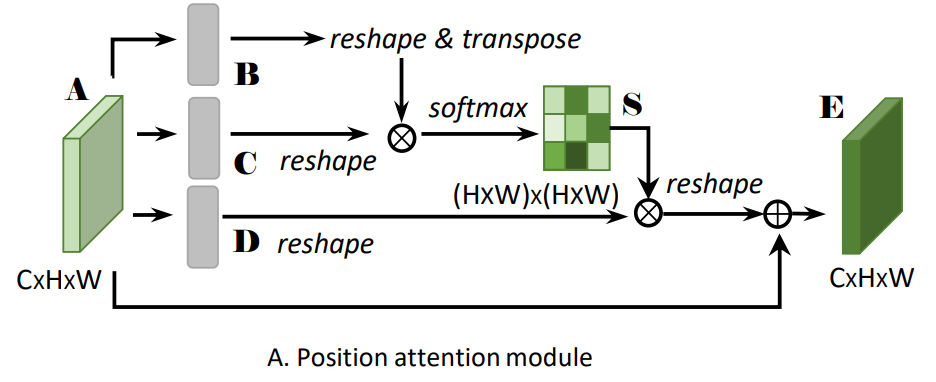代码展示

# 位置注意力
def position_attention(inputs):
# 定义可训练变量，反向传播可更新
gama = tf.Variable(tf.ones(1))  # 初始化1

# 获取输入特征图的shape
b, h, w, c = inputs.shape

# 深度可分离卷积[b,h,w,c]==>[b,h,w,c//8]
x1 = layers.SeparableConv2D(filters=c//8, kernel_size=(1,1), strides=1, padding='same')(inputs)
# 调整维度排序[b,h,w,c//8]==>[b,c//8,h,w]
x1_trans = tf.transpose(x1, perm=[0,3,1,2])
# 重塑特征图尺寸[b,c//8,h,w]==>[b,c//8,h*w]
x1_trans_reshape = tf.reshape(x1_trans, shape=[-1,c//8,h*w])
# 调整维度排序[b,c//8,h*w]==>[b,h*w,c//8]
x1_trans_reshape_trans = tf.transpose(x1_trans_reshape, perm=[0,2,1])
# 矩阵相乘
x1_mutmul = x1_trans_reshape_trans @ x1_trans_reshape
# 经过softmax归一化权重
x1_mutmul = tf.nn.softmax(x1_mutmul)

# 深度可分离卷积[b,h,w,c]==>[b,h,w,c]
x2 = layers.SeparableConv2D(filters=c, kernel_size=(1,1), strides=1, padding='same')(inputs)
# 调整维度排序[b,h,w,c]==>[b,c,h,w]
x2_trans = tf.transpose(x2, perm=[0,3,1,2])
# 重塑尺寸[b,c,h,w]==>[b,c,h*w]
x2_trans_reshape = tf.reshape(x2_trans, shape=[-1,c,h*w])

# 调整x1_mutmul的轴，和x2矩阵相乘
x1_mutmul_trans = tf.transpose(x1_mutmul, perm=[0,2,1])
x2_mutmul = x2_trans_reshape @ x1_mutmul_trans

# 重塑尺寸[b,c,h*w]==>[b,c,h,w]
x2_mutmul = tf.reshape(x2_mutmul, shape=[-1,c,h,w])
# 轴变换[b,c,h,w]==>[b,h,w,c]
x2_mutmul = tf.transpose(x2_mutmul, perm=[0,2,3,1])
# 结果乘以可训练变量
x2_mutmul = x2_mutmul * gama

# 输入和输出叠加
return x

## 3.2 通道注意力模块

通道注意力模块的流程图如下。

（1）分别对特征图A做reshape(CxN)以及reshape与transpose(NxC)；

（2）将得到的两个特征图矩阵相乘tf.multul()，再通过softmax得到归一化后的权重X(C×C)

（3）接着把权重X的转置(CxC)与reshape后的特征图A(CxN)做矩阵乘法tf.multul()，再乘以尺度系数β，再reshape为原来形状。其中β初始化为0，并逐渐的学习得到更大的权重

（4）最后与输入特征图A相叠加得到最后的输出特征图E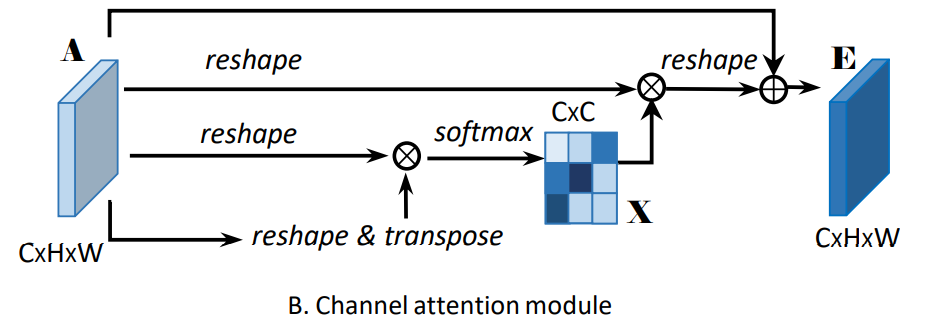代码展示

# 通道注意力
def channel_attention(inputs):
# 定义可训练变量，反向传播可更新
gama = tf.Variable(tf.ones(1))  # 初始化1

# 获取输入特征图的shape
b, h, w, c = inputs.shape

# 重新排序维度[b,h,w,c]==>[b,c,h,w]
x = tf.transpose(inputs, perm=[0,3,1,2])  # perm代表重新排序的轴
# 重塑特征图尺寸[b,c,h,w]==>[b,c,h*w]
x_reshape = tf.reshape(x, shape=[-1,c,h*w])

# 重新排序维度[b,c,h*w]==>[b,h*w,c]
x_reshape_trans = tf.transpose(x_reshape, perm=[0,2,1])  # 指定需要交换的轴
# 矩阵相乘
x_mutmul = x_reshape_trans @ x_reshape
# 经过softmax归一化权重
x_mutmul = tf.nn.softmax(x_mutmul)

# reshape后的特征图与归一化权重矩阵相乘[b,x,h*w]
x = x_reshape @ x_mutmul
# 重塑形状[b,c,h*w]==>[b,c,h,w]
x = tf.reshape(x, shape=[-1,c,h,w])
# 重新排序维度[b,c,h,w]==>[b,h,w,c]
x = tf.transpose(x, perm=[0,2,3,1])
# 结果乘以可训练变量
x = x * gama

# 输入和输出特征图叠加

return x

## 3.3 总体流程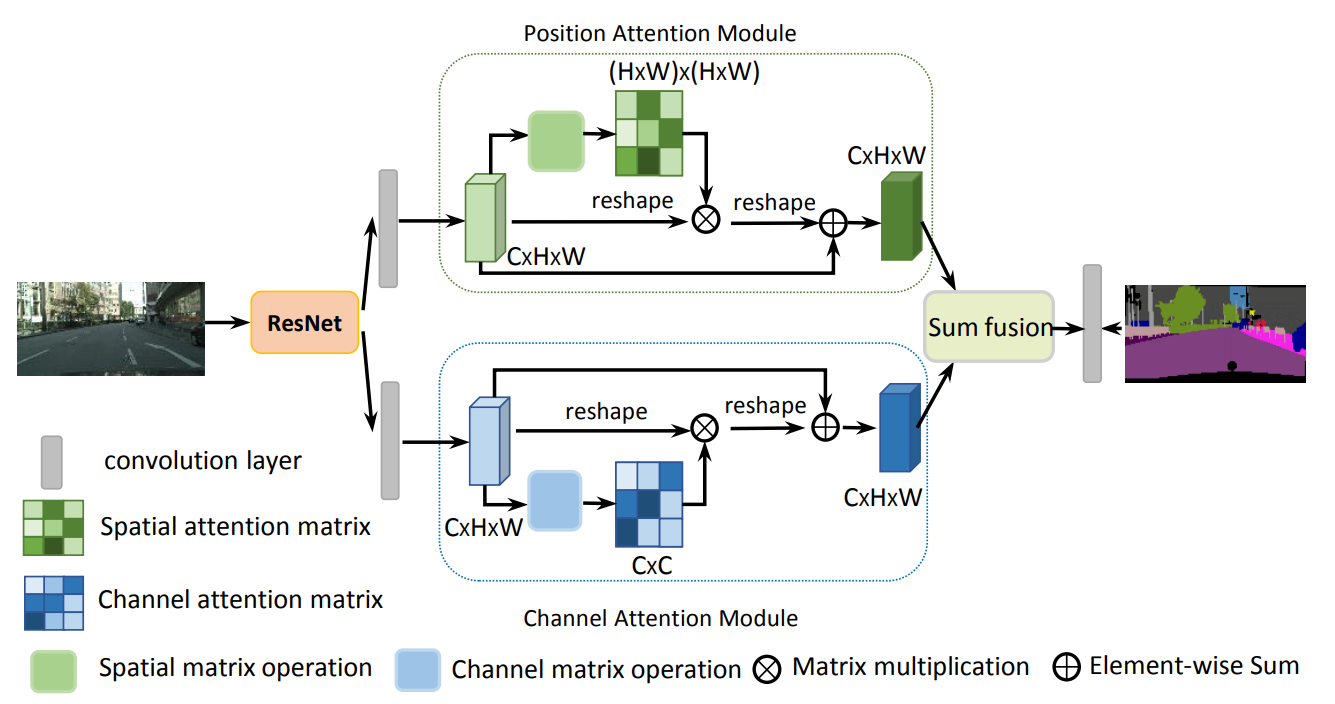完整代码展示

import tensorflow as tf
from tensorflow import keras
from tensorflow.keras import layers, Model

#（1）通道注意力
def channel_attention(inputs):
# 定义可训练变量，反向传播可更新
gama = tf.Variable(tf.ones(1))  # 初始化1

# 获取输入特征图的shape
b, h, w, c = inputs.shape

# 重新排序维度[b,h,w,c]==>[b,c,h,w]
x = tf.transpose(inputs, perm=[0,3,1,2])  # perm代表重新排序的轴
# 重塑特征图尺寸[b,c,h,w]==>[b,c,h*w]
x_reshape = tf.reshape(x, shape=[-1,c,h*w])

# 重新排序维度[b,c,h*w]==>[b,h*w,c]
x_reshape_trans = tf.transpose(x_reshape, perm=[0,2,1])  # 指定需要交换的轴
# 矩阵相乘
x_mutmul = x_reshape_trans @ x_reshape
# 经过softmax归一化权重
x_mutmul = tf.nn.softmax(x_mutmul)

# reshape后的特征图与归一化权重矩阵相乘[b,x,h*w]
x = x_reshape @ x_mutmul
# 重塑形状[b,c,h*w]==>[b,c,h,w]
x = tf.reshape(x, shape=[-1,c,h,w])
# 重新排序维度[b,c,h,w]==>[b,h,w,c]
x = tf.transpose(x, perm=[0,2,3,1])
# 结果乘以可训练变量
x = x * gama

# 输入和输出特征图叠加

return x

#（2）位置注意力
def position_attention(inputs):
# 定义可训练变量，反向传播可更新
gama = tf.Variable(tf.ones(1))  # 初始化1

# 获取输入特征图的shape
b, h, w, c = inputs.shape

# 深度可分离卷积[b,h,w,c]==>[b,h,w,c//8]
x1 = layers.SeparableConv2D(filters=c//8, kernel_size=(1,1), strides=1, padding='same')(inputs)
# 调整维度排序[b,h,w,c//8]==>[b,c//8,h,w]
x1_trans = tf.transpose(x1, perm=[0,3,1,2])
# 重塑特征图尺寸[b,c//8,h,w]==>[b,c//8,h*w]
x1_trans_reshape = tf.reshape(x1_trans, shape=[-1,c//8,h*w])
# 调整维度排序[b,c//8,h*w]==>[b,h*w,c//8]
x1_trans_reshape_trans = tf.transpose(x1_trans_reshape, perm=[0,2,1])
# 矩阵相乘
x1_mutmul = x1_trans_reshape_trans @ x1_trans_reshape
# 经过softmax归一化权重
x1_mutmul = tf.nn.softmax(x1_mutmul)

# 深度可分离卷积[b,h,w,c]==>[b,h,w,c]
x2 = layers.SeparableConv2D(filters=c, kernel_size=(1,1), strides=1, padding='same')(inputs)
# 调整维度排序[b,h,w,c]==>[b,c,h,w]
x2_trans = tf.transpose(x2, perm=[0,3,1,2])
# 重塑尺寸[b,c,h,w]==>[b,c,h*w]
x2_trans_reshape = tf.reshape(x2_trans, shape=[-1,c,h*w])

# 调整x1_mutmul的轴，和x2矩阵相乘
x1_mutmul_trans = tf.transpose(x1_mutmul, perm=[0,2,1])
x2_mutmul = x2_trans_reshape @ x1_mutmul_trans

# 重塑尺寸[b,c,h*w]==>[b,c,h,w]
x2_mutmul = tf.reshape(x2_mutmul, shape=[-1,c,h,w])
# 轴变换[b,c,h,w]==>[b,h,w,c]
x2_mutmul = tf.transpose(x2_mutmul, perm=[0,2,3,1])
# 结果乘以可训练变量
x2_mutmul = x2_mutmul * gama

# 输入和输出叠加
return x

#（3）DANet网络架构
def danet(inputs):

# 输入分为两个分支
x1 = channel_attention(inputs)  # 通道注意力
x2 = position_attention(inputs)  # 位置注意力

# 叠加两个注意力的结果
return x

# 构建网络
if __name__ == '__main__':

# 构造输入层
inputs = keras.Input(shape=[26,26,512])
# 经过DANet注意力机制返回结果
outputs = danet(inputs)

# 构造模型
model = Model(inputs, outputs)
# 查看模型结构
model.summary()

查看网络参数量

Total params: 296,512
Trainable params: 296,512
Non-trainable params: 0
展开全文tensorflow 深度学习 神经网络 python
• 本文提出了一种基于双路细化注意力机制的图像描述模型，该模型同时使用了空间注意力机制与通道注意力机制，并且使用了细化图像特征的模块，对图像特征进行进一步细化处理，过滤掉图像中的冗余与不相关的特征....
• 通过加入空间注意力机制进行单幅图像的去雨，去雾操作
• 一般而言，注意力机制可以分为通道注意力机制，空间注意力机制，以及二者的结合。SENet是通道注意力机制的典型实现。 重点是获得输入进来的特征层对应的每一个通道的权值 。通过学习的方式自动获取每个特征
• 即注意力权重施加的方式和位置不同，将注意力机制分为空间域、通道域和混合域三种，并且介绍了一些关于这些不同注意力的先进注意力模型，仔细分析了他们的的设计方法和应用领域，给出了实现的代码与实验结果。...Attention
• 通道注意力+空间注意力，可嵌入在大部分的主流网络中，在不显著增加计算量和参数量的前提下能提升模型的特征提取能力。CBAM CAM
• 神经网络学习小记录63——Keras 各类注意力机制解析与代码详解学习前言什么是注意力机制 学习前言 注意力机制是一个非常有效的tricks，注意力机制的实现方式有许多，我们一起来学习一下。 什么是注意力机制 ...keras 神经网络 图像处理 Attention
• 参考链接: 深度学习卷积神经网络重要结构之通道注意力和空间注意力模块 参考链接: 用于卷积神经网络的注意力机制(Attention)----CBAM: Convolutional Block Attention Module 参考链接: link 参考链接: link 参考...
• 多头注意力机制 总体描述 这是原文的的图片，我们可以看到，Q，K，V 是三个固定值，分别通过一个Linear层进行映射，Linear层有3个，使用的注意力评分函数为Scaled Dot-Product Attentio ， 有3个代表有3个头，最后...机器学习 深度学习 自然语言处理
• 神经网络学习小记录63——Pytorch 图像处理中注意力机制的解析与代码详解学习前言什么是注意力机制代码下载注意力机制的实现方式1、SENet的实现2、CBAM的实现3、ECA的实现 学习前言 注意力机制是一个非常有效的trick...pytorch 神经网络 图像处理 attention
• 大家好，今天和各位分享一下如何使用 Pytorch 构建卷积神经网络中的各种注意力机制，如：SENet，ECANet，CBAM。注意力机制的原理 和 TensorFlow2 的实现方式可以看我下面两篇博文：SENet、ECANet：...pytorch 深度学习 神经网络
• 缩放点积注意力（Scaled Dot-Product Attention）模块导入遮蔽softmax操作加性注意力代码：补充知识：1.torch.repeat_interleave(data, repeat= , dim=)2.torch.nn.Linear(*in_features*, *out_features*, *bias=...机器学习 算法
• 公众号关注 “Python遇见机器学习” 设为“星标”，第一时间知晓最新干货~ 作者丨pprp 来源丨GiantPandaCV 导读： CBAM模块由于其使用的广泛性以及易于集成得到很多应用。 目前CV领域中的Attention机制也是在20 19年
• U-Net网络模型（简单改进版） 这一段时间做项目用到了U-Net网络模型...***下采样的过程中加入了通道注意力和空间注意力（大概就是这样）*** 代码跑出来后，效果比原来的U-Net大概提升了一个点左右，证明是有效的。 ...计算机视觉 深度学习
• 文章目录一、空间注意力机制简介二、空间注意力与pytorch代码三、使用案例 一、空间注意力机制简介 空间注意力的示意图如下： 长条的是通道注意力机制，而平面则是空间注意力机制，可以发现：通道注意力在意的是每个...pytorch 深度学习 神经网络
• 注意力机制模仿了生物观察行为的内部过程，即一种将内部经验和外部感觉对齐从而增加部分区域的观察精细度的机制。注意力机制可以快速提取稀疏数据的重要特征，因而被广泛用于自然语言处理任务。 当过载信息映入眼帘...python 机器学习 编程语言 自然语言处理 人工智能
• 四 问题思索 《An Empirical Study of Spatial Attention Mechanisms in Deep Networks》 —空间注意力机制 作者：Xizhou Zhu, Dazhi Cheng,etc. 单位：微软亚研 发表会议及时间：arXiv 2019 论文地址 相关代码 ...
• 目录基本概念配置实现源码分析训练效果小结 在上一篇《【YOLOv4探讨 之七】利用... 基本概念 空间注意力机制使用SAM模块，在Darknet中，新添加的sam_layer层就是用于SAM模块，该层在darknet.h中的定义为sam. 其原理计算机视觉 darknet yolov4 YOLOv4
• 本文主要参考开源资料对Attention注意力机制进行总结，注意力机制本质上与人类对外界事物的观察机制相似。以下是相关学习资料推荐： 深度学习中的注意力机制_CSDN大数据-CSDN博客 目前主流的attention方法都有哪些？...深度学习 神经网络 attention
• 相反，人类将注意力集中于局部视觉空间，以便在需要的时间和场合获取信息并对不同时段的信息进行合并，构建内在场景表现，指导日后的注意力分配及决策过程。 《视觉注意循环模型》，2014 本文将探讨如何实施注意...人工智能 ai
• 文章目录一，注意力机制二，自注意力（self-attention）三，软注意力机制1，空域注意力2,通道注意力机制3，混合域模型4，Non-Local5,位置注意力机制四，强注意力机制 一，注意力机制 attention 机制可以认为是一种...计算机视觉
• 空间注意力机制就是学习整个画面不同区域的系数，同时考虑到了所有通道 class ChannelAttention(nn.Module): def __init__(self, in_planes, ratio=16): super(ChannelAttention, self).__init__() self.avg_...深度学习
• 注意力机制 CVPR2021 Coordinate Attention || Pytorch代码实现 即插即用！ 一、Coordinate Attention 简介 二、使用步骤 1.结构图 1.pytorch 代码 即插即用！ 提示：这里可以添加本文要记录的大概内容： ...深度学习 分类算法 python 计算机视觉
• 本文整理了深度学习中最常用的6种注意力机制的数学原理和代码实现。 1、Full Attention 2017的《Attention is All You Need》中的编码器-解码器结构实现中提出。它结构并不复杂，所以不难理解。 上图 1.左侧显示了 ...深度学习 机器学习 神经网络
• ## 注意力机制(Attention Mechanism)

万次阅读 多人点赞 2020-06-25 20:30:30
计算机视觉（computer vision）中的注意力机制（attention）的基本思想就是想让模型学会注意力——能够忽略无关噪声信息而关注重点信息。 自注意力（self-attention） NLP 论文链接：Attention Is All You Need 代码...计算机视觉
• 文章目录题目注意力机制多头注意力机制为什么要使用多头注意力机制 题目 transformer学习之多头注意力机制 注意力机制 详细了解 ➡️ 注意力机制 之前我们也学习过了Seq2Seq，知道了把注意力机制加入到它后会使模型...深度学习 人工智能
• 各模块简介： CBAM 是一个轻量级的通用模块，其中包含了空间注意力和通道注意力。...空间注意力pytorch代码： class SpatialAttention(nn.Module): def __init__(self, kernel_size=7): super(S...深度学习 pytorch...